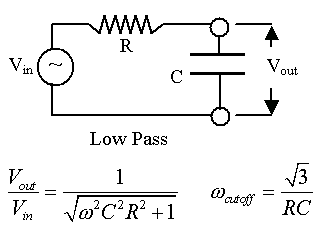### Low-Pass Filter in RC-AC Circuits

 Using a resistor (R) and an capacitor (C) in series with an AC voltage source, we can create a simple low-pass filter. We by changing the R and C values, you can adjust what frequencies get passed at, say, greater than half the original voltage.     There is an illustration of the circuit below. Set the initial parameters of the circuit : R =  ohms C =    microFarads frequency scale factor =  cycles/sec            Here are the equations we will use ...